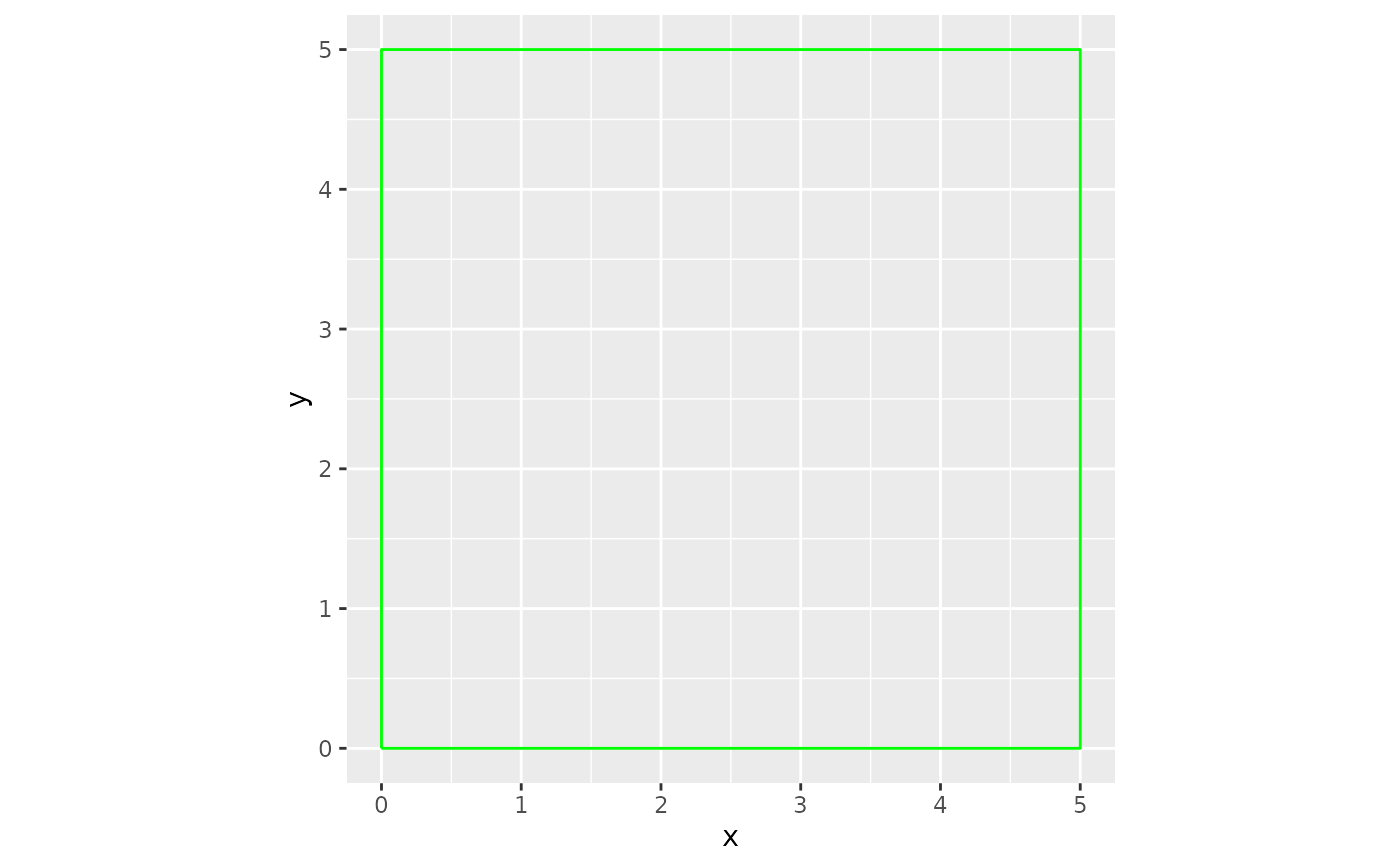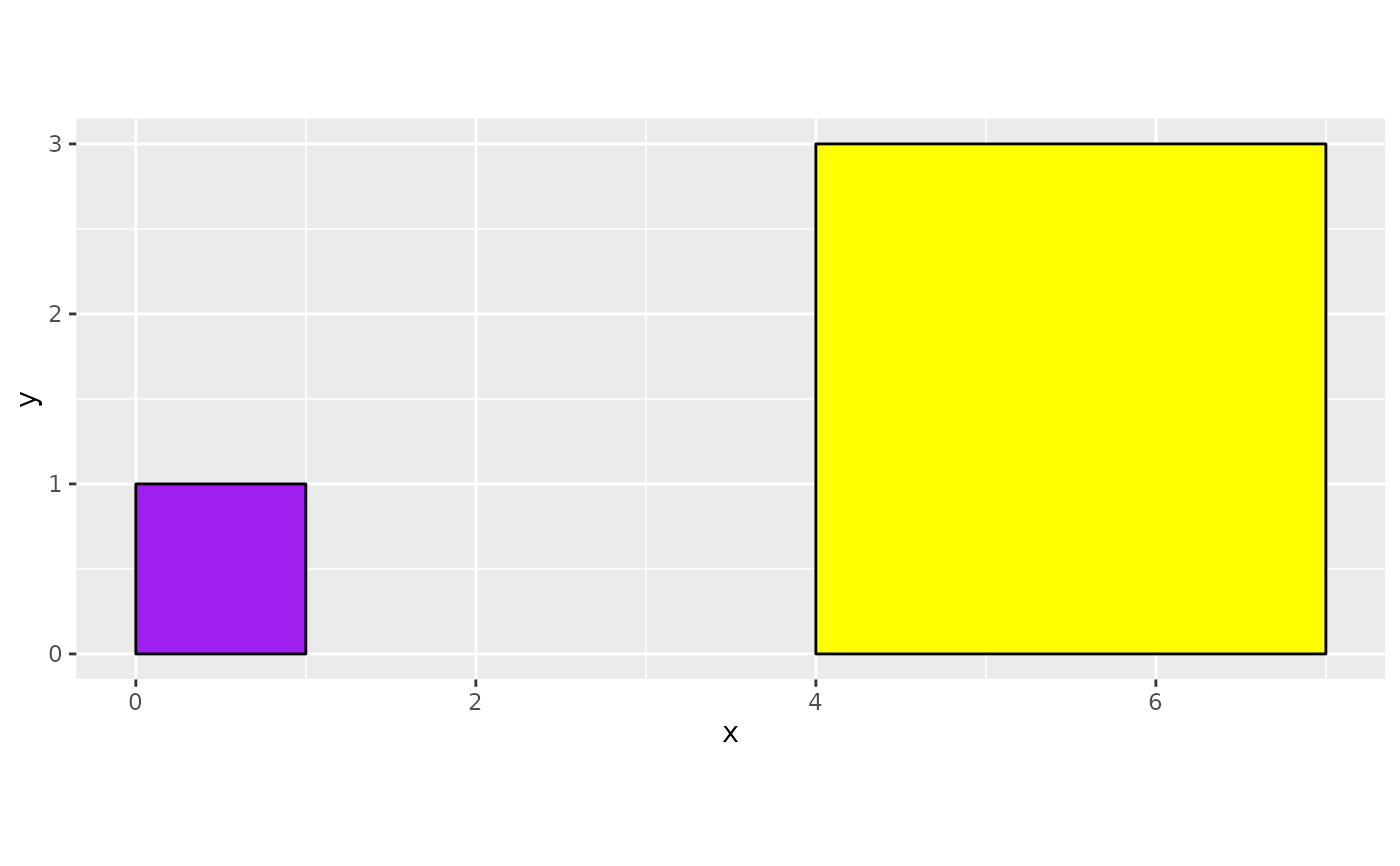A tool for creating a data frame of values that create a square with a specified size when plotted.

The geom_path and geom_polygon geoms are recommended with this data for use in ggplot2 for generative art.

## Usage

square_data(
x,
y,
size,
color = NULL,
fill = NULL,
n_points = 100,
group_var = FALSE,
group_prefix = "square_"
)

## Arguments

x

Numeric value of length 1 - The bottom left x value of the square.

y

Numeric value of length 1 - The bottom left y value of the square.

size

Numeric value of length 1 that must be greater than 0 - The size of the square.

color

Character value of length 1 - The color of the square's border. A valid R color from colors() or a standard 6 digit hexadecimal webcolor like "#000000"

fill

Character value of length 1 - The color of the square. A valid R color from colors() or a standard 6 digit hexadecimal webcolor like "#000000"

n_points

Numeric value. Default is 100. This determines how many points the square will have. This option can come in handy when using jitter options or other texture/illusion methods. Must be of length 1 and at least a value of 4.

group_var

Logical. Default is FALSE. If TRUE, a group variable will be added to the dataframe. Useful in iterative data generation.

group_prefix

Character string of length 1 - The prefix used for the group variable. Default is "square_"

A Tibble

## Examples

# Creating one square
library(ggplot2)
one_square <- square_data(x = 0, y = 0, size = 5)

# Plot The Data
one_square |>
ggplot(aes(x,y))+
geom_path(color = "green")+
coord_equal()# To create multiple squares, use your preferred method of iteration:
# Creating two squares

library(purrr)
library(dplyr)

x_vals <- c(0,4)
y_vals <- c(0,0)
sizes <- c(1,3)
fills <- c("purple", "yellow")
square_n <- 1:2

lst_square_specs <-
list(
x_vals,
y_vals,
sizes,
fills,
square_n
)

# Use square_data() in your preferred iteration methods
two_squares <- pmap(lst_square_specs, ~square_data(
x = ..1,
y = ..2,
size = ..3,
fill = ..4,
color = "#000000",
group_var = TRUE
) |>
# square_data adds a group variable if group_var = TRUE.
# For multiple squares, a unique identifier should be added/pasted in.
mutate(group = paste0(group,..5))
) |>
list_rbind()

# Plot the data

two_squares |>
ggplot(aes(x, y, group = group))+
theme(legend.position = "none")+
geom_polygon(color = two_squares$color, fill = two_squares$fill) +
coord_equal()